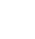# Vlookup in Excel

Vlookup (and its counterpart Hlookup which we deal with later) is an incredibly powerful function in Excel. It’s ability to automate results in a workbook can save lots of time, and provide 100% accuracy when used correctly. It looks quite daunting at first but once the formula logic is broken down into plain English and plain description it should be a bit clearer.

V = Vertical
Lookup = Lookup

As with all formulas in Excel they should be in 1 cell and always start with an = which tells Excel this is going to be a formula to deal with.

By typing =Vlookup you will be presented with a handy help tip which acts as a reminder of where you are up to in the formula as well. If you get stuck, just refer to the handy tip.

The logic of the formula goes between the brackets and we should remember that the Vlookup formulas main ‘anchor’ for it to refer to or deal with is the further most left column of any data range you select. This column is also counted as number 1, the first column in the range. The next column to the right is 2, then 3 and so on. You can use huge numbers of columns if you need to as your ‘area of interest’.

So using the following formula below we can identify the corresponding value in the 2nd column along in the range, to the value we have in D11. It will be exactly the same value as in D11 because we have used FALSE at the end of the logic. (If we were to use TRUE it would find the first closest match to D11 but is not always reliable!).
``` =Vlookup(D11,A:B,2,FALSE) ```
Columns A:B are our ‘area of interest’ and Vlookup will take the value in D11 and work it’s way (extremely quickly) V-Vertically down column A, being the furthermost left and when it finds the same value that is in D11 it stops and then heads off to the right, moving 2 columns, so as it includes the further most left column as 1, column 2 is the next along to the right. In this instance Column B. Whatever value is in column B where it has stopped will be your result.

This image should give you a further idea and uses the same cell references in the examples above.Tips for Vlookup:

You may encounter errors if you try to lookup more columns to the right than you have allowed in the logic. ie using A:C is 3 columns A,B,C but if you try to look in the 4th you will get an error.

It is also possible to encounter errors when vlooking up numbers where a number is the match you are looking for. If you do encounter errors we recommend you contact us for help using the form below, or leave a comment and when we respond, everyone can learn – maybe even us !